## Dynamics Exam1 and Problem Solutions

Dynamics Exam1  and  Problem Solutions

1. A box is pulled with 20N force. Mass of the box is 2kg and surface is frictionless. Find the acceleration of the box.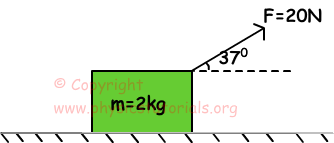We show the forces acting on the box with following free body diagram.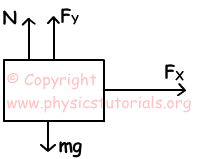X component of force gives acceleration to the box.

FX=F.cos370=20.0,8=16N

FX=m.a

16N=2kg.a

a=8m/s

2. Picture given below shows the motion of two boxes under the effect of applied force. Friction constant between the surfaces is k=0,4. Find the acceleration of the boxes and tension on the rope. (g=10m/s2, sin370=0,6, cos370=0,8)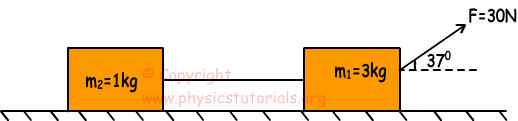Free body diagram of these boxes given below.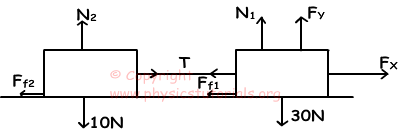Components of force,

FX=F.cos370=30.0,8=24N

FY=F.sin370=30.0,6=18N

N1=m1.g-Fy=30-18=12N

N2=10N

Ff1 and Ff2 are the friction forces acting on boxes.

Ff1=k.N1=0,4.12=4,8N and Ff2=k.N2=0,4.10=4N

We apply Newton's second law on two boxes.

m1: Fnet=m.a

20-T-Ff1=3.a   20-T-4,8=3.a

m2: T-Ff2=1.a  T-4=a

a=2,8m/s2

T=6,8N

3. As you can see in the picture given below, two boxes are placed on a frictionless surface. If the acceleration of the box X is 5m/s2, find the acceleration of the box Y.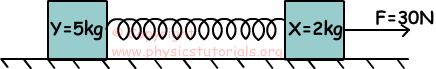Free body diagrams of boxes are given below;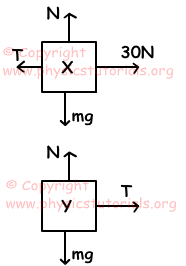Fnet=m.a

(30-T)=2.5

T=20N

Fnet=m.a

T=5.a

20=5.a      a=4m/s2

4. In the system given below ignore the friction and masses of the pulleys. If masses of X and Y are equal find the acceleration of the X?(g=10m/s2)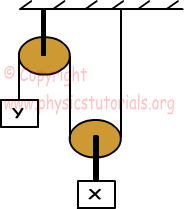Free body diagrams of boxes are given below;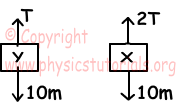Since force acting on X is double of force acting on Y, aX=2aY

For X: 2T-10m=m.a

For Y: T-10m=m.2a

a=2m/s2

5. When system is in motion, find the tension on the rope.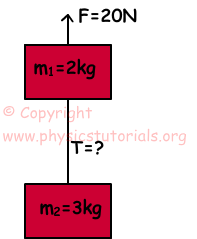Free body diagrams of boxes are given below.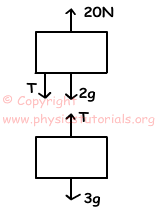m1: T+2g-20=2.a

m2: 3g-T=3.a

5g-20=5.a

a=g-4 putting it into m1 equation;

T+2g-20=2(g-4)

T=12N

Author: